# How to Round Decimals to Decimal Places

How to Round Decimals to Decimal Places• To count decimal places, count the number of digits after the decimal point.
• In 0.5814, 5 is the 1st decimal place, 8 is the 2nd, 1 is the 3rd and 4 is the 4th.
• To round a decimal to a decimal place, first draw a line after the decimal place required.
• So to round 0.5814 to 1 decimal place, we draw a line after the 5.
• We then look at the digit immediately to the right of the line. In this case, this is 8.
• If this digit is 5 or more, we round up and if the digit is 4 or less, we round down.
• To round up, increase the digit before the line by 1 and to round down, keep the digit before the line the same.
• Finally remove all digits after the line.
• In this example, 8 is larger than 4 so we round up.
• We increase the 5 to a 6 and remove all of the other digits after the line.
• 0.5814 rounds up to 0.6 when written to 1 decimal place.
Count the number of decimal places after the decimal point and draw a line to the right of this digit.

If the digit to the right of the line is 5 or more, increase the digit to the left of the line by 1. If the digit is 4 or less, keep this digit the same.

Remove all of the digits after the line.• To round 3.3685 to 3 decimal places, first find the 3rd decimal place.
• The3rd decimal place is the 3rd digit after the decimal point, which is 8.
• We draw a line after this digit and look at the next digit to the right, which is 5.
• If this digit is 5 or more, we round up and if it is 4 or less, we round down.
• 5 rounds up and so, we increase the number to the left of the line by 1 and remove all digits after the line.
• 3.3685 rounds up to 3.369 when written to 3 decimal places.# Rounding to Decimal Places

## How to Round to a Given Number of Decimal Places

To round to a given number of decimal places, use these steps:

1. Count the same number of digits after the decimal point as the number of decimal places being rounded to.
2. Draw a vertical line immediately after this digit.
3. If the digit to the right of this line is 5 or more, increase the digit to the left of the line by 1.
4. If the digit to the right of this line is 4 or less, keep the digit to the left of the line the same.
5. Remove all digits that come after the line.

For example, round 2.8397 to 3 decimal places.

The first step is to count 3 digits after the decimal point to find the 3rd decimal place. This is the digit of 9.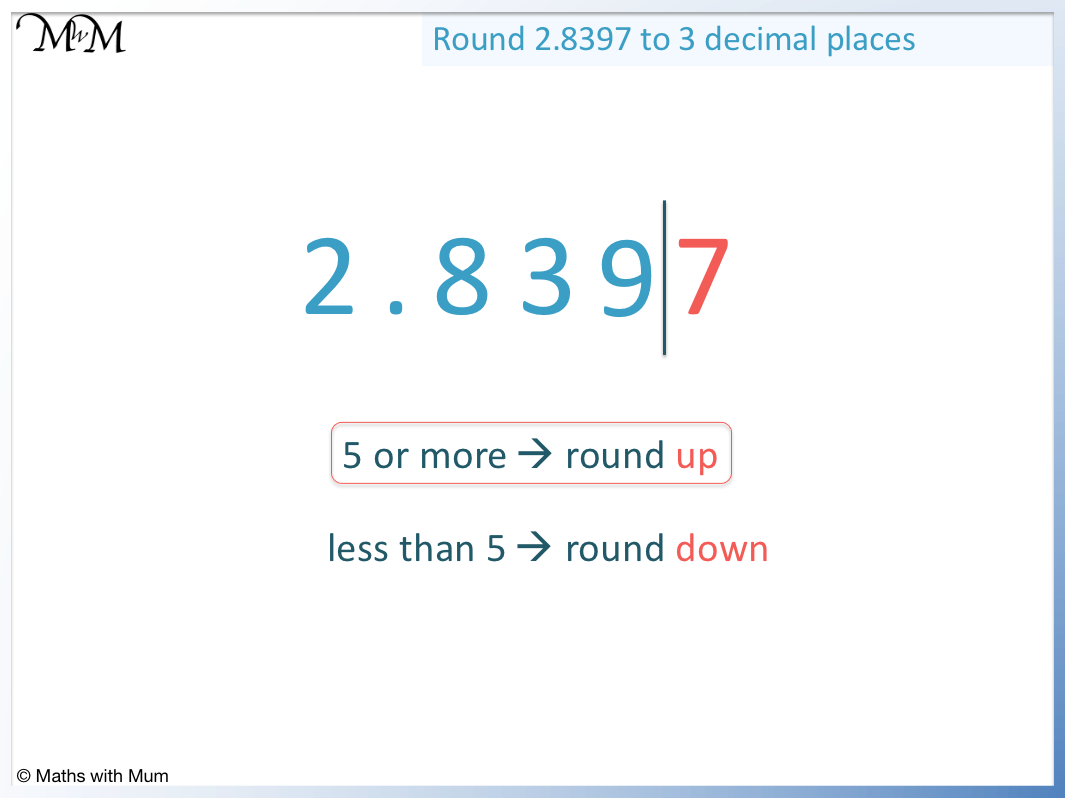The second step is to draw a line after the 9.

The 3rd step is to look at the digit to the right of this line, which is a 7.

7 is ‘5 or more’ and so, it rounds this number up.

We increase the digit to the left of the line by 1. Since this digit is a 9, we look at the digit in front of this as well. We look at the digits of 3 and 9 in 2.8397 and increase 39 to 40.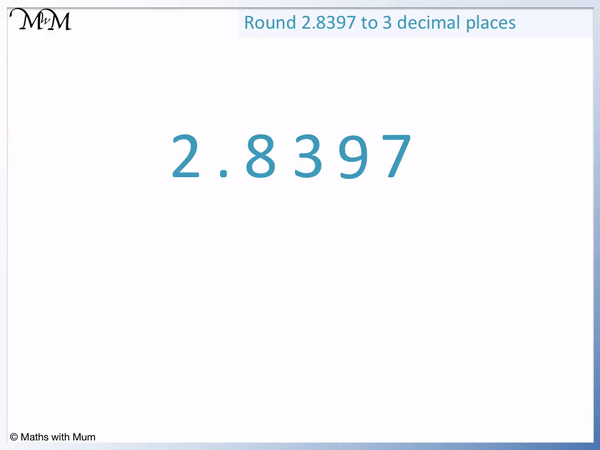We then remove all digits from after the line.

2.8397 rounds up to 2.840 when written to 3 decimal places.

## How to Round to the Nearest Tenth

To round to the nearest tenth, look at the 2nd digit after the decimal point (the hundredths column). If this digit is 5 or more, increase the 1st digit after the decimal point (the tenths column) by 1. If the hundredths digit is 4 or less, keep the tenths digit the same. Finally, remove all digits after the tenths column.

Rounding to the nearest tenth means the same as rounding to 1 decimal place.

This is because the tenths column is the first digit after the decimal point.

For example, round 0.5814 to the nearest tenth. The choice is to round up to 0.6 or to round down to 0.5.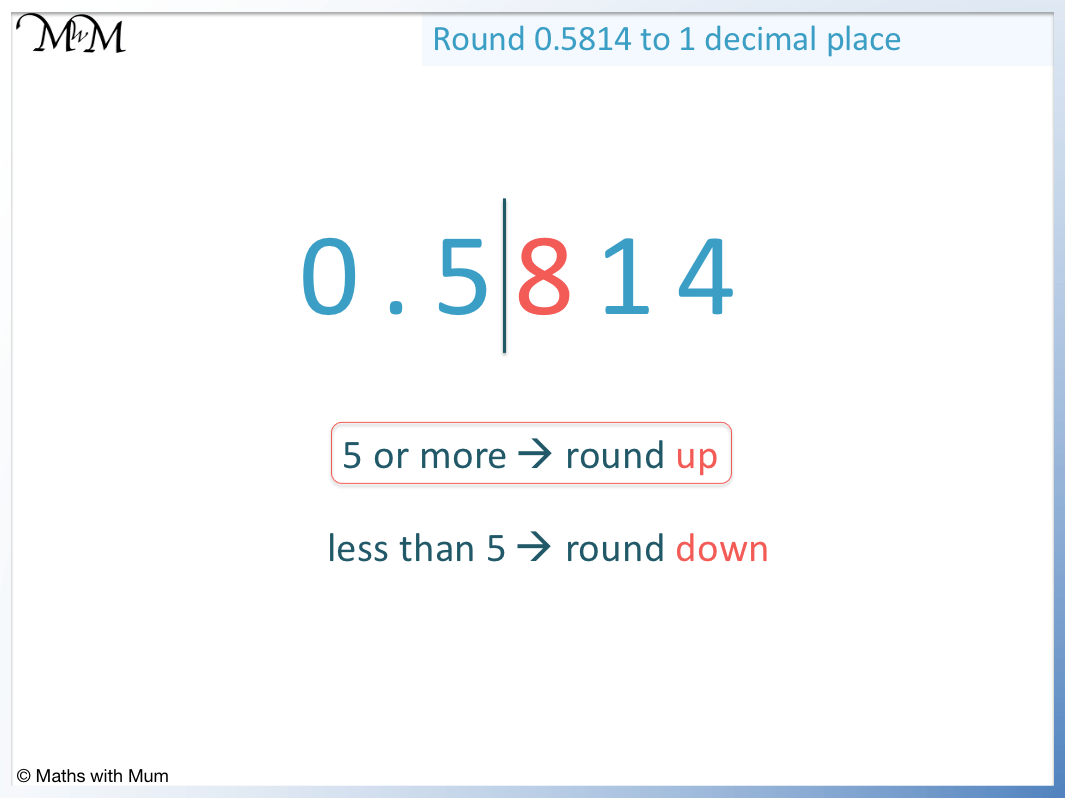The first step is to look at the 2nd digit after the decimal point.

In this example, this is an 8.

The next step is to decide whether to round up or down depending on whether this digit is 5 or more or 4 or less.

8 is in the ‘5 or more’ category and so, we round up.

To round up, increase the tenths digit by 1 and remove the digits after the tenths column.

0.5814 rounds up to 0.6 when rounded to the nearest tenth.Here are some examples of rounding to 1 decimal place (to the nearest tenth).

Number Rounded to the Nearest Tenth
0.43 0.4
0.48 0.5
0.25 0.3
1.6723 1.7
8.6174 8.6
7.4499 7.4

Notice that if the digit in the 2nd decimal place is a 5, we still round up. For example, 0.25 round up to 0.3.

## How to Round to the Nearest Hundredth

To round to the nearest hundredth, look at the 3rd digit after the decimal point (the thousandths column). If this digit is 5 or more, increase the 2nd digit after the decimal point (the hundredths column) by 1. If the thousandths digit is 4 or less, keep the hundredths digit the same. Finally, remove all digits after the hundredths column.

Rounding to the nearest hundredth means the same as rounding to 2 decimal places.

For example, round 1.9021 to the nearest hundredth. The 2nd decimal place is 0 so the choice is to round up to 1.91 or down to 1.90.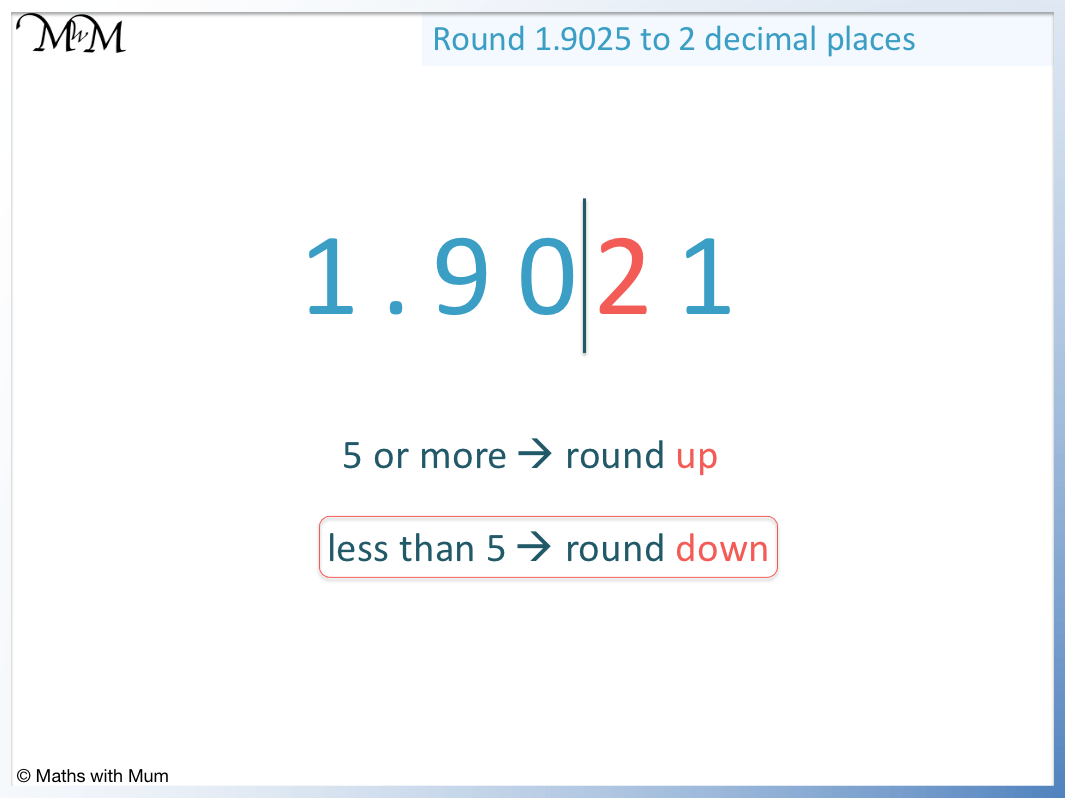The first step is to look at the digit in the 3rd decimal place, which is the 2.

The next step is to decide whether to round up or down depending on whether this digit is 5 or more or 4 or less.

2 is in the ‘4 or less’ category and so, rounds down.

To round down, keep the hundredths digit the same and remove the digits after this.

The hundredths digit is 0 and so, we keep this the same and remove the 2 and the 1 from after it.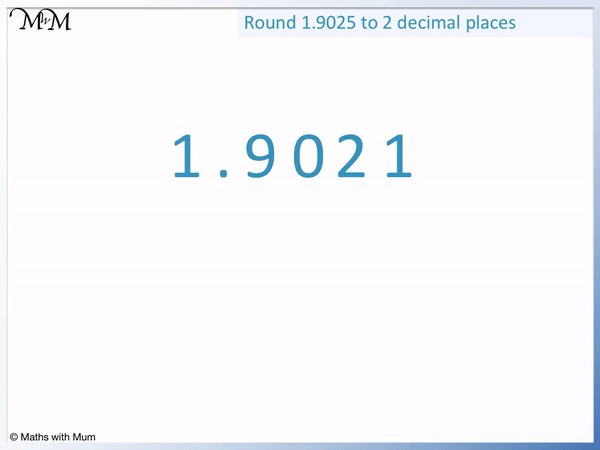Here are some examples of rounding to 2 decimal places.

Number Rounded to the Nearest Hundredth
0.621 0.61
0.627 0.63
0.625 0.63
5.1718 5.17
4.3071 4.31
2.34499 2.34
9.396 9.40

## How to Round to the Nearest Thousandth

To round to the nearest thousandth, look at the 4th digit after the decimal point (the ten-thousandths column). If this digit is 5 or more, increase the 3rd digit after the decimal point (the thousandths column) by 1. Instead, if this digit is 4 or less, keep the thousandths digit the same. Finally, remove all digits after the thousandths column.

Rounding to the nearest thousandth means the same as rounding to 3 decimal places.

For example, round 3.3685 to the nearest thousandth. The 3rd digit after the decimal point is 8 and so the choice is to round up to 3.369 or down to 3.368.The first step is to look at the 4th digit after the decimal point, which is 5.

If this digit is 5 or more, round up and if it is 4 or less, round down.

5 rounds up.

Therefore the 3rd digit in the hundredths column is increased from 8 to 9 and 3.3685 rounds up to 3.369.

Here are some examples of rounding to 3 decimal places.

Number Rounded to the Nearest Thousandth
0.7332 0.733
0.7339 0.734
0.7335 0.734
8.02384 8.024
1.21912 1.219
3.02449 3.024
6.8596 6.860

## Why do we Round Decimals?

The purpose of rounding decimals is to make a number easier to work with or to read. The further a digit is after the decimal point, the less value it contributes to the overall size of the number. Therefore, removing later digits does not significantly change the value of the number. For example, instead of writing 3.14159, it can be easier to say that this number is approximately 3 or 3.1.

Rounding shortens the length of a decimal.

For example, 3.3685 contains 4 digits after the decimal point. Rounding this number to 3 decimal places we get 3.369, which only has 3 digits after the decimal point.Many decimal numbers can go on for a very long time, containing lots of decimal places. Some decimal numbers are called irrational numbers and they go on forever.

For example, pi is an irrational number. The first few digits of pi are 3.14159. However, it would be impossible to read the entire number because it goes on forever. For example, adding one more decimal place we get 3.141592 and then we get 3.1415926, and so on.

At some point, with irrational numbers, we have to cut them off at some stage and rounding tells us the rules for doing this accurately.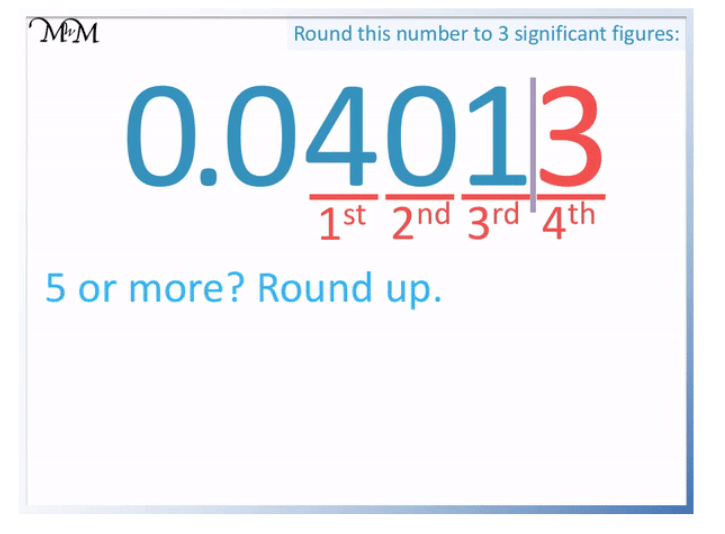Now try our lesson on Rounding to Significant Figures where we learn what significant figures are.